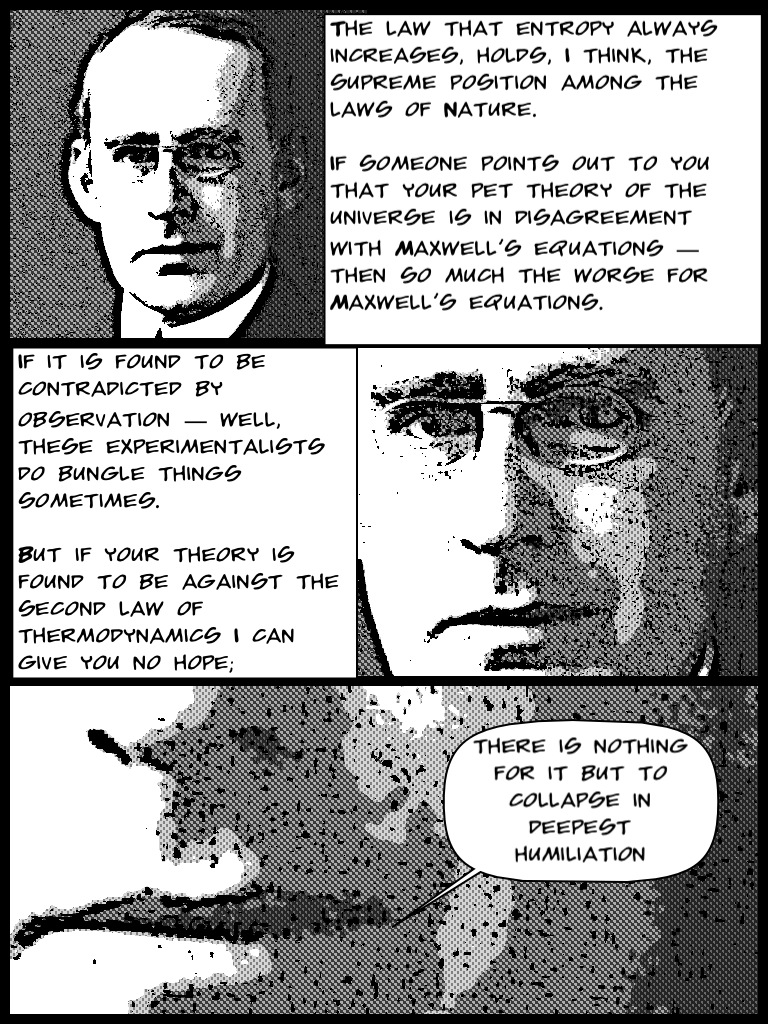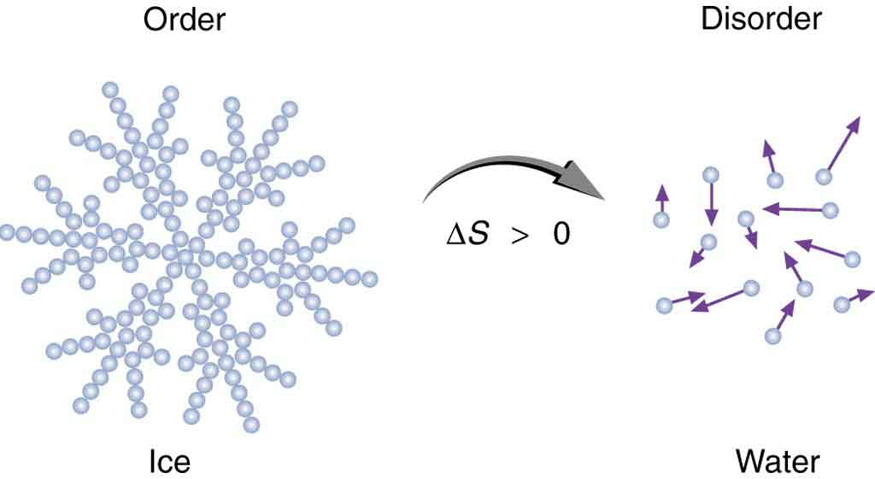#It’s About Heat and Temperature

What is the difference between heat and temperature?  Heat is thermal energy.  Temperature is a measurement of the average kinetic energy of the particles which compose the matter being tested.  When heat flows into a material, one of two things happen: either the temperature of the material can rise, or there may be a change in its state (such as from ice to liquid, or liquid to vapour).

The difference is heat is thermal energy transferred from one object to another because of a temperature difference, and temperature is a relative measure of how hot or cold something is measured on a scale, or the average kinetic energy of the particles in a substance or object.

In the simplest of cases, if a small amount of heat Q is transferred gently to a body, whilst the temperature of the body is T, the entropy of the body increases by Q/T.

### Whenever heat is transferred to a body, the entropy increases.$\Delta S = \frac {Q}{T}$

# The Entropy of a Steak (or Veggie Burger) on a Plate

When heat flows between a steak (or veggie burger) and a plate there is no violation of energy conservation: the energy lost by the steak is gained by the plate.  However, conservation of energy does not explain why the heat always flows from the hot steak to the cold plate. This is where the second law of thermodynamics comes in.

## Second Law of Thermodynamics

The total entropy S of an isolated system cannot decrease.  In general, entropy does increase.$\Delta S > 0$

Suppose the steak is at temperature T, the plate is at a slightly lower temperature 0.95T, and that a small amount of heat Q is transferred from the steak to the plate.

The entropy of the steak then decreases by Q/T while the entropy of the plate increases by Q/0.95T.  It is easy to see that the entropy lost by the steak is smaller than the entropy gained by the plate, so the total entropy of the Universe has increased.  This process is therefore consistent with the second law of thermodynamics.  On the other hand, if heat Q had flowed from the cold plate to the hot steak, the entropy lost by the plate (Q/0.95T) would have been greater than the entropy gained by the steak (Q/T), and the total entropy of the Universe would have decreased.This violates the second law of thermodynamics, so we can be sure that the process is impossible.  Heat flow is said to be an irreversible process – you will NEVER see heat flowing spontaneously from a cold body to a hotter one.

# The Entropy of H2O

This post begins with a picture representing the entropy a melting snowflake.

Let’s now look at a quantitative example…

## When Ice Melts…

1.00 kg of ice (pure H2O at 0°C) placed in a bucket is allowed to melt gradually while remaining at standard atmospheric pressure.  Under such conditions, the specific latent heat of melting ice is$3.33 \times 10^5 J kg^{-1}$.

How much will the entropy of H2O have increased when the ice has all turned into liquid water, while its temperature is still 0°C?

The heat transferred to ice in order to melt it at a fixed temperature is the product of the mass of ice and its specific latent heat of melting.$Q_{rev} = 1.00 kg \times 3.33 \times 10^5 J kg^{-1} = 3.33 \times 10^5 J$.

A temperature of 0°C is equivalent to T = 273.15 K.

The change in entropy for ice melting is then$\Delta S = \frac {Q_{rev}}{T} = \frac {3.33 \times 10^5 J}{273 K} = 1.22 \times 10^3 J K^{-1}$.

## When Water Evaporates…

1.00 kg of boiling water (pure H2O at 100°C) placed in a kettle is converted into steam.  Under these conditions, the relevant specific latent heat of vaporisation is$2.26 \times 10^6 J kg^{-1}$.

How much will the entropy of H2O change when the water has been converted into steam at the same temperature of 100°C?

The heat transferred to liquid water in order to evaporate it at a fixed temperature is the product of the mass of water and its specific latent heat of vaporisation.$Q_{rev} = 1.00 kg \times 2.26 \times 10^6 J kg^{-1} = 2.26 \times 10^6 J$.

A temperature of 100°C is equivalent to T = 373.15 K.

The change in entropy for water evaporating is then$\Delta S = \frac {Q_{rev}}{T} = \frac {2.26 \times 10^6 J}{373 K} = 6.06 \times 10^3 J K^{-1}$.